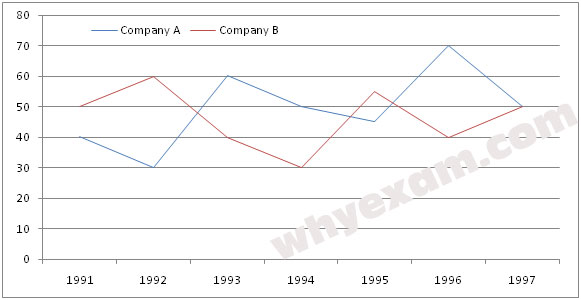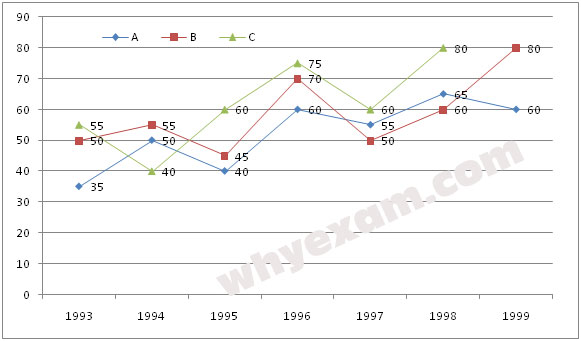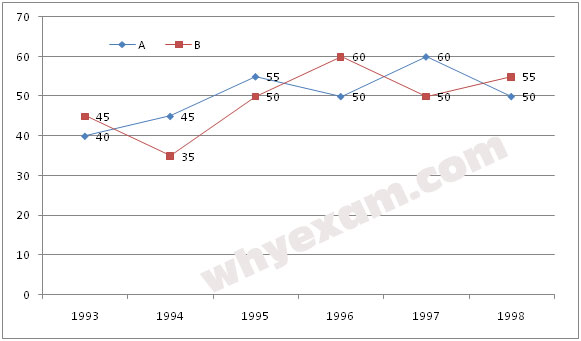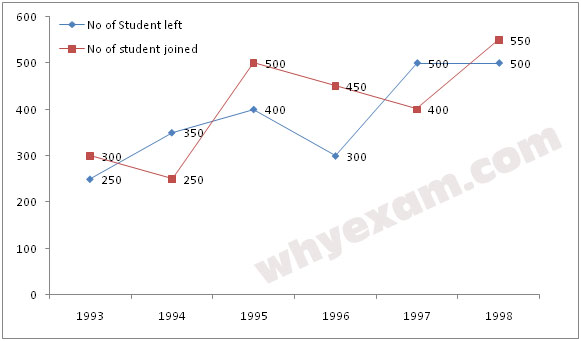# Line Graph Questions and Answers

## About Line Graph in Data Interpretation

Line Graph is another representation of data. It may be consider the another form of Bar Chart. Line graph represents performance over time. Line Chart represents trend, cycle and data against a time period.

Generally here X axis represent time period and Y axis represent variable data in respect to time. Time period may be year, month, days, hours etc. Line chart is very important for measurement of economic and business performance.

Normally questions are asked in Data Interpretation form Line Graph section are mentioned below

1. Growth of company over the year
2. Growth of population of any country, state or area
3. Volume of export and import of any company
4. GDP of any country

## Questions and Answers on Line Graph Data Interpretation

Directions (1-5): Study the following graph carefully and answers the following questions below.

Percentage of net profit of two companies over the years1. If the total income in 1992 for Company B was 140 crores, what was the total expenditure in that year?
A. 100 crores
B. 110 crores
C. 98 crores
E. None of these

2. If the total expenditure of 1993 and 1994 together of Company B was Rs 270 crores, what was the total income in these years?
A. Rs 121.5 crores
B. Rs 135 crores
C. Rs 140 crores
E. None of these

3. In how many given years the percentage of expenditure to the income of Company A was less than fifty?
A. One
B. Two
C. Three
D. Four
E. None of these

4. If the total expenditure of Company in 1994 was Rs 200 crores, what was the total income?
A. Rs 160 crores
B. Rs 240 crores
C. Rs 260 crores
E. None of these

5. In which of the following years was the total income more than doubles the total expenditure in that year for Company B?
A. 1995
B. 1993
C. 1997
D. 1992
E. None of these

## Data Interpretation Model Questions and Answers on Line Graph

Directions (6-10): Study the following graph carefully and answer the questions given below it.

Production of sugar (in thousand tons) by three different sugar factories over the years.6. For which of the following pairs of years the total production of three companies together is equal?
A. 1993-94
B. 1995-97
C. 1996-98
D. 1994-95

7. In which of the following years company A the percent rise/fall from the previous year is the maximum?
A. 1996
B. 1993
C. 1995
D. 1998
E. None of these

8. Average production per year for Company B is approximately what percent of the average production per year for company C?
A. 105%
B. 85%
C. 107%
D. 93%
E. 97%

9. What is the percentage rise in production of company C in 1995 to 1996?
A. 20%
B. 22%
C. 18%
D. 15%
E. None of these

10. What is the difference between the average production of the three companies together in 1997 and the average production of the three companies together in 1999 in thousand tons?
A. 20
B. 15
C. 17
D. 22
E. None of these

Directions (11-15): Read the graph carefully and answer the questions given below it.11. The income of Company B in the year 1994 was Rs 10800. How much was expenditure in that year?
A. Rs 900000
B. Rs 80000
C. Rs 185000
D. Rs 195000
E. None of these

12. Company A spends Rs 350000 in the year 1995. Find out the income of Company A in that year.
A. Rs 1542500
B. Rs 1525000
C. Rs 1525800
D. Rs 1233000
E. None of these

13. If the expenditure of both the Company A and B in the year 1997 was equivalent, then what was the ratio between the incomes of Company A to Company B?
A. 15:6
B. 6:15
C. 16:5
D. 15:5
E. None of these

14. In which of the following year was there maximum percentage of growth/decline with respect to the previous year in case of Company B?
A. 1997-96
B. 1994-94
C. 1996-97
D. 1995-96
E. None of these

15. The expenditure of Company A in the year was Rs 250000 and the income of 1995 was the expenditure in the year 1996. Find out the income in the year 1996.
A. Rs 13,87,5000
B. Rs 15,12,500
C. Rs 15,12,250
D. Rs 15,87,500
E. None of these

## Sample Questions and Answers on Line Graph

Directions (16-20): Read the graph carefully and answer the questions given below it.

Percent profit earned by two companies A and B over the year16. If income for company A in the year 1994 was 35 lakhs what was the expenditure for company B in the same year?
A. 123.5 lakhs
B. 128 lakhs
C. 132 lakhs
E. None of these

17. The income of company A in 1996 and the income of company B in 1997 are equal. What will be the ratio of expenditure of company A in 1996 to the expenditure of company B in 1997?
A. 26:7
B. 37:6
C. 15:170
D. 116:17
E. None of these

18. During which of the following years the ratio of percent profit earned by company A to that of company B was the maximum?
A. 1993 & 1996 both
B. 1995 & 1997 both
C. 1993 only
D. 1998 only
E. None of these

19. If the expenditure of company B increased by 20% from 1995 to 1996, the income in 1996 will be how many times the income 1995?
A. 2.16 times
B. 1.2 times
C. 1.8 times
D. Equal
E. None of these

20. If the income of company A in 1996 was Rs 36 lakhs, what was the expenditure of company A in 1996?
A. 122.5 lakhs
B. 128.8 lakhs
C. 120 lakhs
D. 121.6 lakhs
E. None of these

Directions (21-25): Read the graph carefully and answer the questions given below it.

The number of students who joined and left the school in the beginning of year for six years, from 1993 to 1998.

Initial strength of the school in 1992=150021. What was the increase/decrease in strength of the school from 1994 to 1995?
A. Increase by 100
B. Decrease by 100
C. Increase by 200
D. Decrease by 200
E. None of these

22. For which of the following years, the percentage rise/fall in number of students left from the previous year is the highest?
A. 1994
B. 1995
C. 1996
D. 1997
E. 1998

23. How many students were there n the school during the year 1996?
A. 1495
B. 1600
C. 1550
D. 1700
E. None of these

24. During which of the following pairs of years, the strengths of the school is equal?
A. 1994 and 1995
B. 1995 and 1997
C. 1996 and 1998
D. 1995 and 1998
E. 1993 and 1995

25. The number of students in 1996 is approximately what percent of the number of students in 1994?
A. 85
B. 117
C. 95
D. 103
E. 108

What is an example of a line graph?

Line graph is the organized presentation of data over a time period. Time period may me day, month year etc. For example you can draw a line chat of daily sale of a store for one month period. Here X axis represents day and Y axis represents amount of sale.

How do you interpret a line graph?

In line graph we draw a horizontal scale and a vertical scale. Here horizontal scale represents time period and vertical scale represent data respect to time period.

What is double line graph?

Double line graph usually used when you compare between two figures. Suppose you want to compare growth of two companies over the last 10 years. Then you need to draw two lies for each company. If you read our example, you will get idea on it.